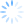Login/Register Math Worksheets, Activities, Games Helpline 099826-99306

# Math worksheet on subtraction of 2 digit numbers

In this subtraction worksheet, students apply the skill in a real life setting. Where is subtraction most naturally used? In finding distances .... Think about it, kids use subtraction even without knowing it in such setting. Print out this colorful math worksheet and give it to them to practice subtraction of 2 digit numbers.Subtraction skill has different levels. For example, the most basic is picture subtraction. The next level is subtraction of 1 digit number from another 1 digit number. Gradually the complexity is increased to subtraction of 2 digit numbers without carry over and then to subtraction with carry over and so on. A further complexity is added by the layout of the problem. For example, vertical subtraction i.e. numbers on top of each other is traditionally easier as compared to horizontal subtraction i.e. different layout etc. These are different skills. Below we have a math worksheet on horizontal subtraction of 2 digit numbers.

For more math worksheets - math worksheets for grade 1, math worksheets on subtraction. Start enjoying math.